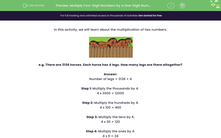# Multiply Four-Digit Numbers by a One-Digit Number

In this worksheet, students will use a column method to multiply four-digit numbers by a one-digit numberKey stage:  KS 2

Curriculum topic:   Number: Multiplication and Division

Curriculum subtopic:   Multiply Numbers to Four Digits

Difficulty level:#### Worksheet Overview

In this activity, we will learn about the multiplication of two numbers.Example

There are 3,136 horses. Each horse has 4 legs. How many legs are there altogether?

Number of legs = 3,136 × 4

Step 1: Multiply the thousands by 4.

4 x 3,000 = 12.000

Step 2: Multiply the hundreds by 4.

4 x 100 = 400

Step 3: Multiply the tens by 4.

4 x 30 = 120

Step 4: Multiply the ones by 4.

4 x 6 = 24

12,000 + 400 + 120 + 24 = 12,544

 × 3 1 3 6 4 4 × 3000 1 2 0 0 0 4 × 100 4 0 0 4 × 30 1 2 0 4 × 6 2 4 1 2 5 4 4

So there are 12,544 legs altogether.

That's a lot of legs!Let's have a go at some questions now.

### What is EdPlace?

We're your National Curriculum aligned online education content provider helping each child succeed in English, maths and science from year 1 to GCSE. With an EdPlace account you’ll be able to track and measure progress, helping each child achieve their best. We build confidence and attainment by personalising each child’s learning at a level that suits them.

Get started